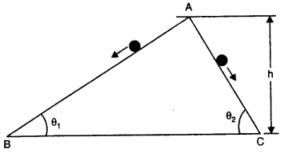# Chapter 6 – Work Energy and power Questions and Answers: NCERT Solutions for Class 11 Physics

Class 11 Physics NCERT book solutions for Chapter 6 - Work Energy and power Questions and Answers.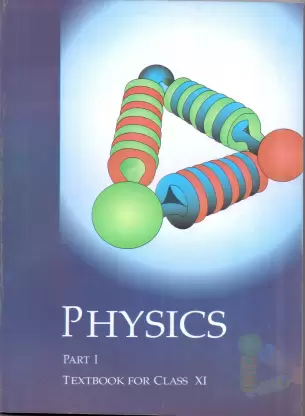## Question 6.3. Given figures are examples of some potential energy functions in one dimension. The total energy of the particle is indicated by a cross on the ordinate axis. In each case, specify the regions, if any, in which the particle cannot be found for the given energy. Also, indicate the minimum total energy the particle must have in each case. Think of some physical contexts for which these potential energy shapes are relevant.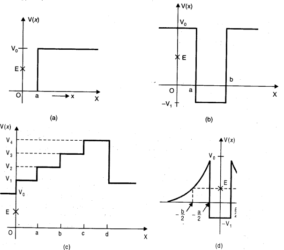## Question 6.4.The potential energy function for a particle executing linear simple harmonic motion is given by V (x) – kx2/2, where k is the force constant of the oscillator. For k = 0.5 Nm-1, the graph of V (x) versus x is shown in Fig. Show that a particle of total energy 1 J moving under this potential must ‘turn back’ when it reaches x = ± 2 m.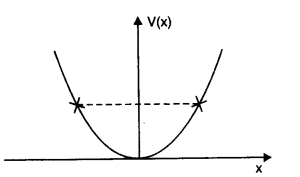### Answer:Here, force constant k = 0.5 Nm-1and total energy of particle E = 1J. The particle can go up to a maximum distance xm, where its total energy is transformed into elastic potential energy.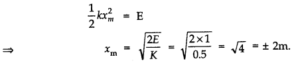## (d) In Fig.(i), the man walks 2 m carrying a mass of 15 kg on his hands. In Fig. (ii), he walks the same distance pulling the rope behind him. The rope goes over a pulley, and a mass of 15 kg hangs at its other end. In which case is the work done greater?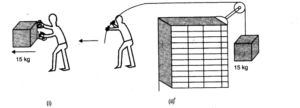## Question 6. 10. A body is moving unidirectionally under the influence of a source of constant power. Its displacement in time t is proportional to(i) t1/2(ii) t (iii) t3/2(iv) t2

### Answer:(ii)p = force x velocity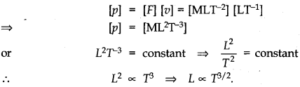## Question 6. 11. A body constrained to move along the z-axis of a coordinate system is subject to a constant forceF given by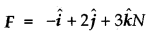## where i, j, k, are unit vectors along the x- y- and z-axis of the system respectively. What is the work done by this force in moving the body a distance of 4 m along the z-axis?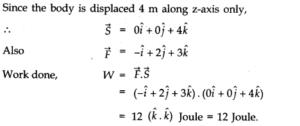## Question 6. 12. An electron and a proton are detected in a cosmic ray experiment, the first with kinetic energy 10 keV, and the second with 100 keV. Which is faster, the electron or the proton? Obtain the ratio of their speeds, (electron mass = 9.11 x 10-31kg, proton mass = 1.67 x 10-27kg, 1 eV= 1.60 x 1019J).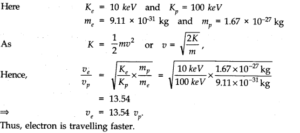## Question 6. 14. A molecule in a gas container hits a horizontal wall with speed 200 msA and angle 30° with the normal, and rebounds with the same speed. Is momentum conserved in the collision? Is the collision elastic or inelastic?

### Answer:Let us consider the mass of the molecule be m and that of wall be M. The wall remains at rest due to its large mass. Resolving momentum of the molecule along x-axis and y-axis, we get The x-component of momentum of molecule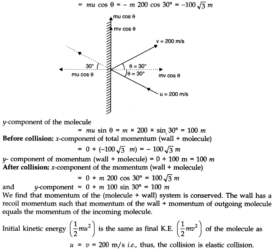## Question 6. 16. Two identical ball bearings in contact with each other and resting on a friction less table are hit head-on by another ball bearing of the same mass moving initially with a speed V. If the collision is elastic, which of the following (Fig.) is a possible result after collision?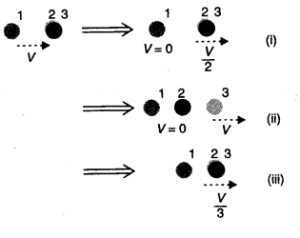## Question 6.17. The bob A of a pendulum released from 30° to the vertical hits another bob B of the same mass at rest on a table as shown in Fig. How high does the bob A rise after the collision? Neglect the size of the bobs and assume the collision to be elastic.

### Answer:Since collision is elastic therefore A would come to rest and B would begin to move with the velocity of A.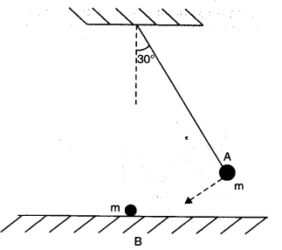## Question 6. 18. The bob of a pendulum is released from a horizontal position. If the length of the pendulum is 1.5 m, what is the speed with which the bob arrives at the lowermost point, given that it dissipated 5% of its initial energy against air resistance?

### Answer:On releasing the bob of pendulum from horizontal position, it falls vertically downward by a distance equal to length of pendulum i.e., h = l = 1.5 m . As 5% of loss in P.E. is dissipated against air resistance, the balance 95% energy is transformed into K.E. Hence,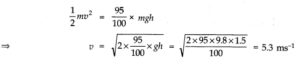## Question 6. 21. The blades of a windmill sweep out a circle of area A. (a) If the wind flows at a velocity v perpendicular to the circle, what is the mass of the air passing through it in time t? (b) What is the kinetic energy of the air? (c) Assume that the windmill converts 25% of the wind’s energy into electrical energy, and that A = 30 m2, v = 36 km/h and the density of air is 1.2 kg m-3. What is the electrical power produced?

### Answer:(a) Volume of wind flowing per second = Av Mass of wind flowing per second = Avρ Mass of air passing in second = Avρt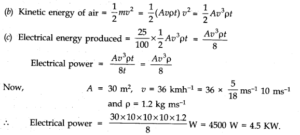## Question 6. 24. A bullet of mass 0.012 kg and horizontal speed 70 ms-1strikes a block of wood of mass 0.4 kg and instantly comes to rest with respect to the block. The block is suspended from the ceiling by thin wire. Calculate the height to which the block rises. Also, estimate the amount of heat produced in the block.

### Answer:Here, m1= 0.012 kg, u1= 70 m/s m2= 0.4 kg, u2= 0 As the bullet comes to rest with respect to the block, the two behave as one body. Let v be the velocity acquired by the combination. Applying principle of conservation of linear momentum, (m1 + m2) v = m1H1 + m2u2 = m1u1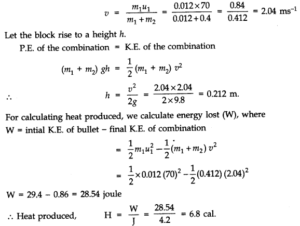## Question 6. 25. Two inclined frictionless tracks, one gradual and the other steep meet at A from where two stones are allowed to slide down from rest, one on each track (Fig). Will the stones reach the bottom at the same time? Will they reach there at the same speed? Explain. Given θ1= 30°, θ2= 60°, and h = 10 m, what are the speeds and times taken by the two stones?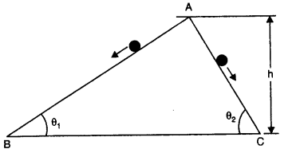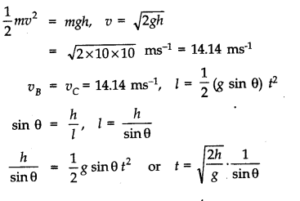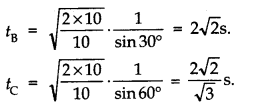## Question 6. 26. A 1 kg block situated on a rough incline is connected to a spring with spring constant 100 Nm-1as shown in Figure. The block is released from rest with the spring in the unstretched position. The block moves 10 cm down the incline before coming to rest. Find the coefficient of friction between the block and the incline. Assume that the spring has negligible mass and the pulley is frictionless.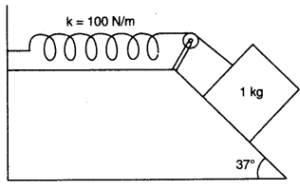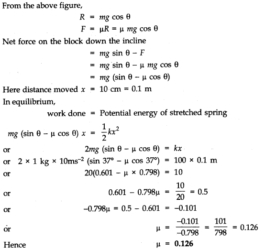## Question 6.29. Which of the following potential energy curves in Fig. cannot possibly describe the elastic collision of two billiard balls? Here r is distance between centres of the balls.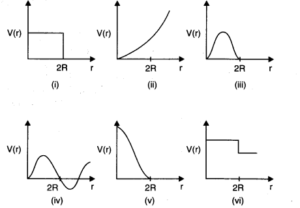## Question 6. 30. Consider the decay of a free neutron at rest: n > p + e–. Show that the two body decay of thistype must necessarily give an electron of fixed energy, and therefore, cannot account for the observed continuous energy distribution in theβ -decay of a neutron or a nucleus, Fig.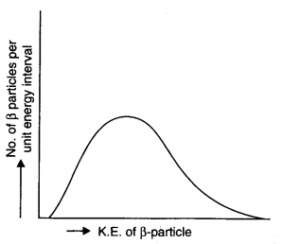### Answer:Let the masses of the electron and proton be m and M respectively. Let v and V be the velocities of electron and proton respectively. Using law of conservation of momentum. Momentum of electron + momentum of proton= momentum of neutron